# Problem

We are given head, the head node of a linked list containing unique integer values.

We are also given the list G, a subset of the values in the linked list.

Return the number of connected components in G, where two values are connected if they appear consecutively in the linked list.

Example 1:

Input:
G = [0, 1, 3]
Output: 2
Explanation:
0 and 1 are connected, so [0, 1] and  are the two connected components.


Example 2:

Input:
G = [0, 3, 1, 4]
Output: 2
Explanation:
0 and 1 are connected, 3 and 4 are connected, so [0, 1] and [3, 4] are the two connected components.


Note:

• If N is the length of the linked list given by head1 <= N <= 10000.
• The value of each node in the linked list will be in the range [0, N - 1].
• 1 <= G.length <= 10000.
• G is a subset of all values in the linked list.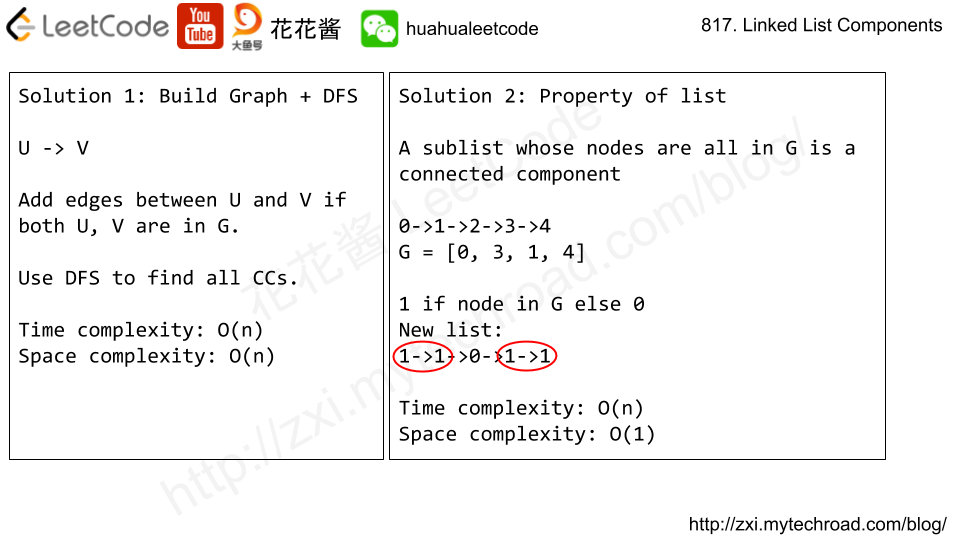# Problem

We have a grid of 1s and 0s; the 1s in a cell represent bricks.  A brick will not drop if and only if it is directly connected to the top of the grid, or at least one of its (4-way) adjacent bricks will not drop.

We will do some erasures sequentially. Each time we want to do the erasure at the location (i, j), the brick (if it exists) on that location will disappear, and then some other bricks may drop because of that erasure.

Return an array representing the number of bricks that will drop after each erasure in sequence.

Example 1:
Input:
grid = [[1,0,0,0],[1,1,1,0]]
hits = [[1,0]]
Output: 
Explanation:
If we erase the brick at (1, 0), the brick at (1, 1) and (1, 2) will drop. So we should return 2.
Example 2:
Input:
grid = [[1,0,0,0],[1,1,0,0]]
hits = [[1,1],[1,0]]
Output: [0,0]
Explanation:
When we erase the brick at (1, 0), the brick at (1, 1) has already disappeared due to the last move. So each erasure will cause no bricks dropping.  Note that the erased brick (1, 0) will not be counted as a dropped brick.

# Idea

1. For each day, hit and clear the specified brick.
2. Find all connected components (CCs) using DFS.
3. For each CC, if there is no brick that is on the first row that the entire cc will drop. Clear those CCs.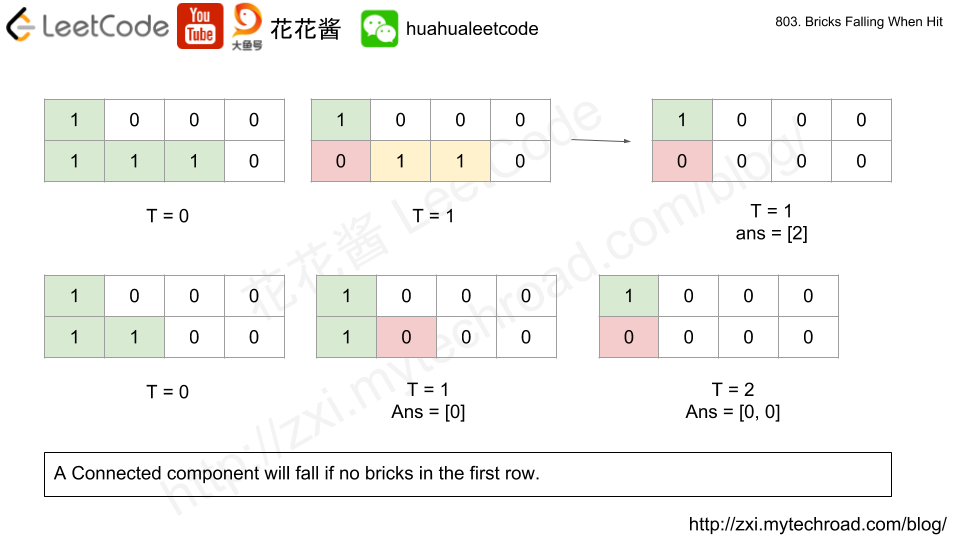# Related Problems

https://leetcode.com/problems/redundant-connection/description/

Problem:

In this problem, a tree is an undirected graph that is connected and has no cycles.

The given input is a graph that started as a tree with N nodes (with distinct values 1, 2, …, N), with one additional edge added. The added edge has two different vertices chosen from 1 to N, and was not an edge that already existed.

The resulting graph is given as a 2D-array of edges. Each element of edges is a pair [u, v] with u < v, that represents an undirected edge connecting nodes u and v.

Return an edge that can be removed so that the resulting graph is a tree of N nodes. If there are multiple answers, return the answer that occurs last in the given 2D-array. The answer edge [u, v] should be in the same format, with u < v.

Example 1:

Example 2:

Note:

• The size of the input 2D-array will be between 3 and 1000.
• Every integer represented in the 2D-array will be between 1 and N, where N is the size of the input array.

Idea:
DFS / Union-Find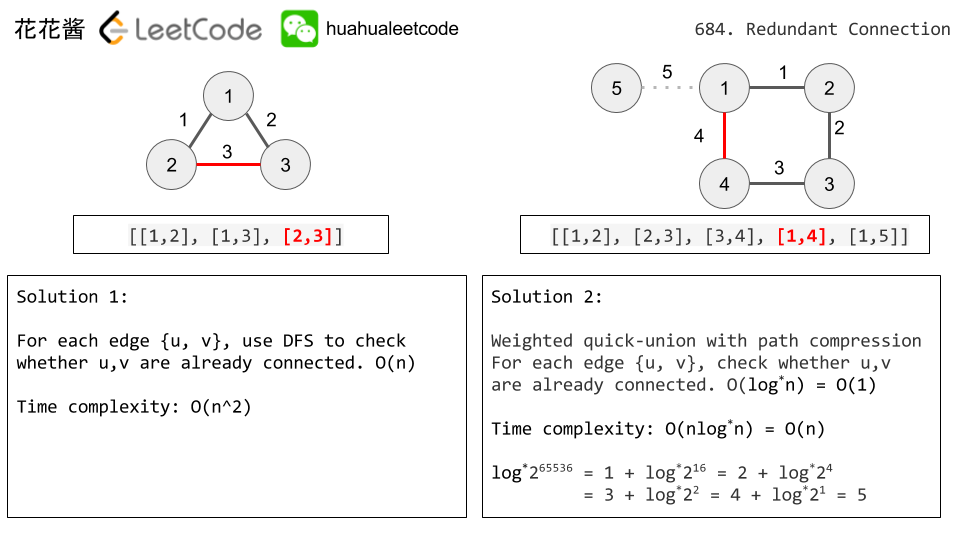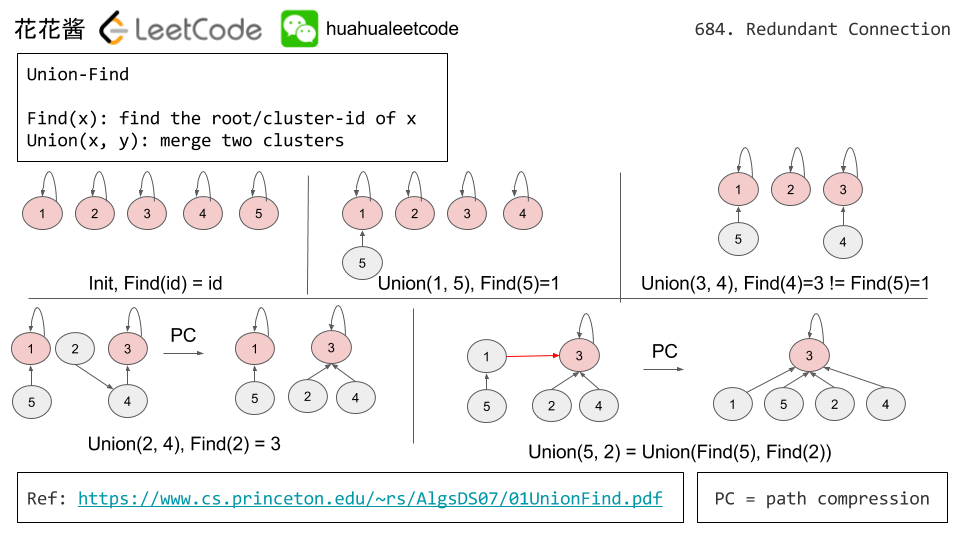Solutions:

C++ / DFS

C++ / Union Find

Java / Union Find

Python: Union Find

Python / Union Find V2

Problem:

There are N students in a class. Some of them are friends, while some are not. Their friendship is transitive in nature. For example, if A is a direct friend of B, and B is a direct friend of C, then A is an indirect friend of C. And we defined a friend circle is a group of students who are direct or indirect friends.

Given a N*N matrix M representing the friend relationship between students in the class. If M[i][j] = 1, then the ithand jth students are direct friends with each other, otherwise not. And you have to output the total number of friend circles among all the students.

Example 1:

Example 2:

1. N is in range [1,200].
2. M[i][i] = 1 for all students.
3. If M[i][j] = 1, then M[j][i] = 1.

Idea:

Find all connected components using DFS

# Related Problems

Problem:

Given a 2d grid map of '1's (land) and '0's (water), count the number of islands. An island is surrounded by water and is formed by connecting adjacent lands horizontally or vertically. You may assume all four edges of the grid are all surrounded by water.

Example 1:

Example 2:

# Idea: DFS

Use DFS to find a connected component (an island) and mark all the nodes to 0.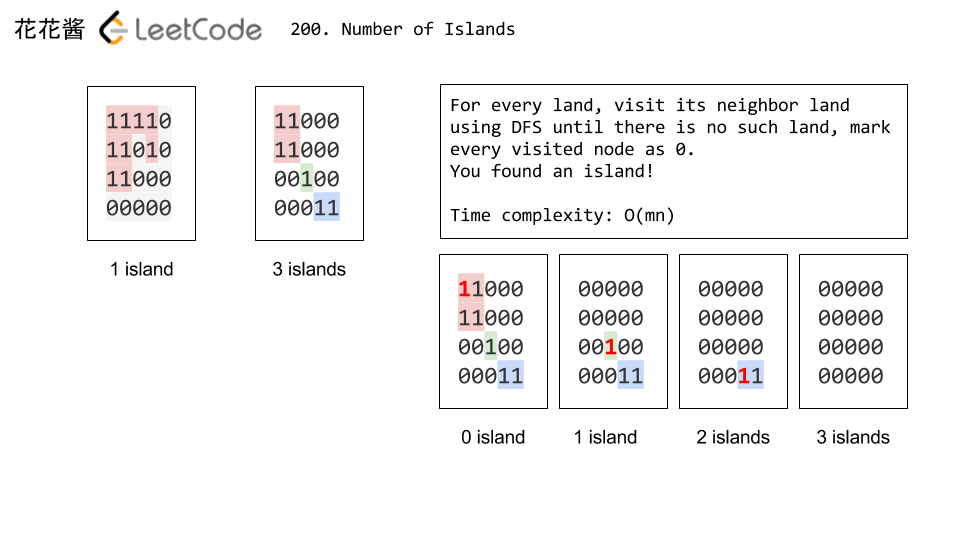Time complexity: O(mn)

Space complexity: O(mn)

# Related Problems

Mission News Theme by Compete Themes.